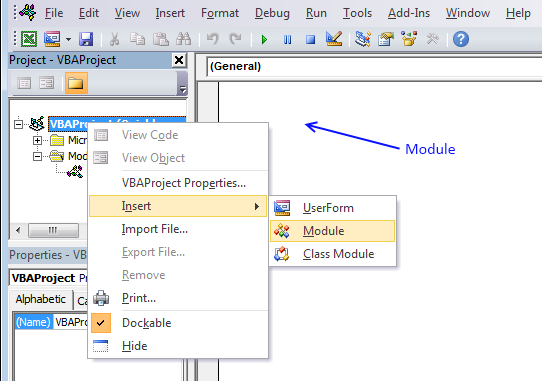Author: Oscar Cronquist Article last updated on January 18, 2019The User Defined Function demonstrated in the above picture extracts unique distinct values also considering lower and upper case letters.

A User defined Function in Excel is a custom function that anyone can use, simply copy the code to your workbook and you are good to go, see details below.

Array formula in cell D3:D10:

=CSUnique(B3:B10)

To enter an array formula, type the formula in a cell then press and hold CTRL + SHIFT simultaneously, now press Enter once. Release all keys.

The formula bar now shows the formula with a beginning and ending curly bracket telling you that you entered the formula successfully. Don't enter the curly brackets yourself.

Have you read the article that extracts unique distinct values (case sensitive) using an array formula?

CSUnique(rng)

### Arguments

 Parameter Text rng Required. The range you want to use.

### VBA

```'Name function and argument
Function CSUnique(rng As Range)
'Declare variables and data types
Dim cell As Range, temp() As String, i As Single, iRows As Integer
'Redimension array variable so it can grow using Redim Preserve statement
ReDim temp(0)
'Iterate through each cell in range
For Each cell In rng
'Iterate through values in array variable temp
For i = LBound(temp) To UBound(temp)
'If value is equal to cell value
If temp(i) = cell Then
i = i + 1
'Stop For ... Next statement
Exit For
End If
Next i
'Subtract variable i with 1
i = i - 1
'If value in array variable temp is not equal to cell value
If temp(i) <> cell Then
'Save cell value to array variable temp
temp(UBound(temp)) = cell
'Add another container to array variable temp
ReDim Preserve temp(UBound(temp) + 1)
End If
Next cell
'Count how many cells have been used when entering UDF
'To prevent error value the UDF adds blanks to remaining containers
If iRows < UBound(temp) Then
temp(iRows - 1) = "More values.."
Else
For i = UBound(temp) To iRows
ReDim Preserve temp(UBound(temp) + 1)
temp(UBound(temp)) = ""
Next i
End If
'Return array variable temp to worksheet
CSUnique = Application.Transpose(temp)
End Function
End Function
```

### Where to copy vba code?1. Press Alt-F11 to open visual basic editor
2. Press with right mouse button on on your workbook in 'Project Explorer' window
3. Press with left mouse button on 'Insert'
4. Press with left mouse button on 'Module'
5. Copy above VBA code
6. Paste VBA code to the code module
7. Exit visual basic editor

### Get the Excel fileFilter-case-sensitive-unqiue-distinct-values.xls